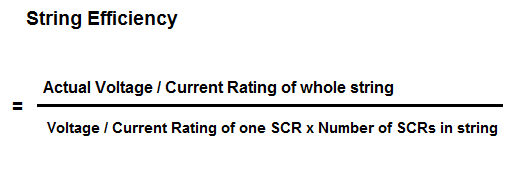# String Efficiency and Derating Factor of SCR

### String Efficiency of SCR:

String Efficiency of SCR is the degree of capacity utilization of individual SCRs in a string of series / parallel connected SCRs. String efficiency is always less than 1.Series or parallel connection of SCR is required to meet the higher voltage / current requirement in industrial application. Though the rating of individual SCR has greatly increased and SCR with voltage and current rating of 10 kV & 3 kA are readily available, but in industrial application the voltage and current requirement may be more. In such case, the SCRs are connected in series to meet the increased voltage requirement whereas it is connected in parallel to fulfill the increased current requirement. But whatever is the fashion of connection, it should be ensured that SCR rating is fully utilized.

As can be seen from the formula of String Efficiency, the value of string efficiency is always less than unity. This means that, the overall voltage across the string is always less than the calculated voltage across the string by considering the individual SCR’s voltage / current rating and number of SCR. This is because the V-I characteristics of SCRs are not identical, therefore unequal voltage / current sharing by the individual SCRs is bound to occur in a string. However, the unequal voltage / current sharing can be minimized by the use of external equalizer ring. But to be honest, the use of equalizer ring, do not make string efficiency unity rather it improves its value.

Let us consider an example to better understand the concept of string efficiency. Figure below shows a string having 4 SCRs. Each SCR is rated foe 600 V and the voltage across the terminals of string is 2100 V.Therefore,

String Efficiency of SCR

=2100 / [600×4]

= 0.875

= 87.5%

### Derating Factor (DFR) of SCR:

Derating Factor is the amount by which the string efficiency deviates from unity or 100%.

DFR = [1 – String Efficiency]

= [100 – % String Efficiency]

Derating Factor gives an idea of unused capacity available in a sting of SCRs. This is the reason; it is a measure of reliability of string. The lesser the value of sting efficiency, the lesser will be voltage / current sharing by the individual SCRs. This means, more unused capacity will be available with the string and hence more DFR. But lesser string efficiency increases the cost of string. Thus a compromise is made in between economy and reliability by properly designing a value of string efficiency.

For our example, the value of derating factor,

DFR = [100 – 87.5%]

= 12.5 %

### One thought on “String Efficiency and Derating Factor of SCR”

1.Rahul Patil says:

All the electrical engineering related imforamation

This site uses Akismet to reduce spam. Learn how your comment data is processed.